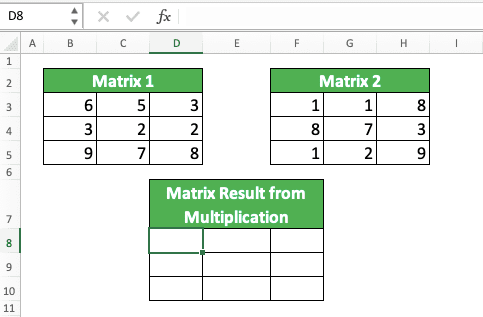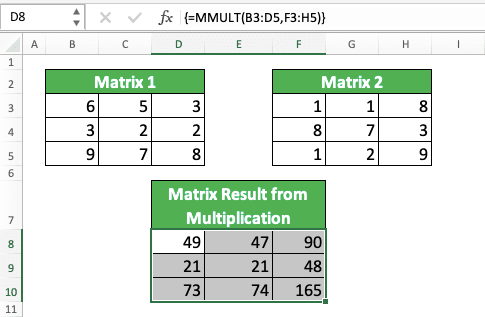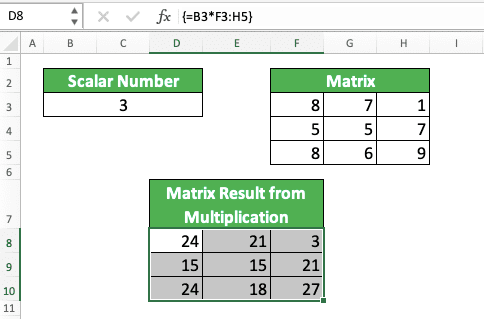How to Do Matrix Multiplication in Excel - Compute Expert

# How to Do Matrix Multiplication in Excel

Home >> Excel Tutorials from Compute Expert >> Excel Calculations >> How to Do Matrix Multiplication in Excel

In this tutorial, you will learn how to do matrix multiplication in excel properly.

When working with numbers in excel, we sometimes have some numbers in the form of a matrix that we must multiply. To get a correct matrix multiplication result, we cannot do the multiplication process as the way we multiply normal numbers. We must do it by using a special matrix multiplication method that excel has provided for us.

Want to know what is the method and how to implement it properly in excel? Read this tutorial until its last part!

Disclaimer: This post may contain affiliate links from which we earn commission from qualifying purchases/actions at no additional cost for you. Learn more

## What is Matrix Multiplication?

Before we dig into how we multiply matrices in excel, let’s discuss a bit about the matrix multiplication itself. What is it and what are its differences with a normal number multiplication?

Matrix multiplication is a multiplication process that tries to find the product of number arrays in columns and rows. We call the number array in columns and rows a matrix and its form look like this (can be in any number of rows and columns).In matrix multiplication, we multiply the rows’ numbers of our first matrix with the columns’ numbers of the second matrix. We sum the multiplication results of each row and column to get our multiplication result in the form of a matrix.

For much easier understanding, see the following matrix multiplication process example. Let’s say we want to multiply these two matrices.How do we do it? Well, as we discussed previously, we multiply the first matrix rows numbers with the second matrix columns numbers. After that, we sum the multiplication results from each row and column.

Thus, to get the top left result of this multiplication, we do a calculation process like this.

= 3x2 + 7x5

The numbers we multiply are from the first row of the first matrix and the first column of the second matrix. We get 41 from the calculation and we put it in the top-left position of the result matrix.

We do this kind of calculation process to get all the numbers in the result matrix. For the top right, here is the calculation (the first row of the first matrix and the second column of the second matrix).

= 3x2 + 7x8

For the bottom left, the calculation is like this (the second row of the first matrix and the first column of the second matrix).

= 8x2 + 4x5

For the bottom right, the calculation is like this (the second row of the first matrix and the second column of the second matrix).

= 8x2 + 4x8

As a result, from the matrix multiplication, we get this matrix.Because the process and the result are like that, there are two points that a matrix multiplication should adhere to.
• The number of columns in the first matrix must be the same as the number of rows in the second matrix
• The matrix result will have the same number of rows as the first matrix. It will also have the same number of columns as the second matrix

We should pay attention to these points when we multiply our matrices in excel so we can run the process correctly.

## Matrix Multiplication in Excel: The MMULT Function

Now that we understand matrix multiplication, how can we do it in excel?

To do a matrix multiplication process in excel, you should use the MMULT function. It is a special function that excel provides to help us multiply matrices.

Here is the general writing form of the MMULT function in excel.

{ = MMULT ( matrix_cell_range1 , matrix_cell_range2 ) }

Why do we have curly brackets around the MMULT formula writing? That is because we should use an array formula form when using MMULT.

After all, we expect its result in an array (matrix). The curly brackets are the symbol of an array formula form in excel.

To write MMULT itself, we just need to input the two matrices we want to multiply. We can input the matrices by using cell ranges coordinates.

After we finish writing the MMULT formula, we press Ctrl + Shift + Enter buttons to invoke the array formula form (we cannot type the curly brackets ourselves to invoke it).

We should also highlight the cell range where we want to put our matrix multiplication result too before we write MMULT. This is so we can get the multiplication result in full, in the form of a matrix.

If we don’t use an array formula form or highlight the cell range first, we will only get one multiplication result (the top left one).

To better understand the MMULT implementation in excel, follow this example. Let’s say we have these two matrices we want to multiply in excel.How to do the multiplication process? Well, first, we highlight the cell range where we want to put the matrix multiplication result like this.Then, we write our MMULT formula. We input the first matrix cell range and the second matrix cell range in our MMULT.To finish, we press Ctrl + Shift + Enter buttons. That will give MMULT an array formula form and thus, we will get the result we want from MMULT!Not too hard, isn’t it?

## Matrix Multiplication with a Scalar Number in Excel

What if you want to multiply your matrix with a scalar number in excel? Is there any way to do this?

Sure, there is. You just need to multiply the number and the matrix normally. However, you need to use an array formula form so you can get the result in full.

Here is the general writing form of the multiplication formula in excel.

{ = scalar_number * matrix_cell_range }

Simple, isn’t it? Just don’t forget to highlight the cell range where you want to put the result first before you write the formula. Don’t forget to also press Ctrl + Shift + Enter buttons after you finish your formula writing.

Here is its implementation example in excel.## Exercise

After you have learned how to multiply matrices in excel, let’s do an exercise to deepen your understanding.

### Questions

1. What is the result if you multiply matrix 1 with matrix 2?
2. What is the result if you multiply matrix 1 with matrix 3?
3. What is the result if you multiply 5 with the no. 1 answer matrix?

If you have any non-numeric value in the matrices you multiply with MMULT, you will get #VALUE errors. Therefore, make sure the cell ranges you input into MMULT contain only numbers in their cells!

Related tutorials you should learn too:

Get updated excel info from Compute Expert by registering your email. It's free!